Select Page

Introduction:

### Quadratic Equations is one of the most important topics that features in CAT Algebra. Consisting of problems that are based on pure algebraic concepts, it requires you to have a deep understanding of the topic and also couple it with extensive practice. In order to master this topic, you need to make sure you solve a fair number of quadratic equation problems from this topic.

Question 1: If the products of the roots of the equation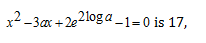then the roots are real for a = ……..
(a) 3
(b) -3
(c) 3 or -3
(d) 0

Question 2: If one root is square of the other root of the equation x2 +ax+b then the relation between a and b is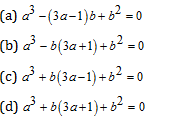Question 3: If p, q, r are the sides of a triangle ABC such that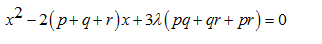has real roots, then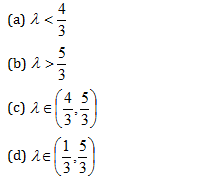Option d

The equation x2 –px +r = 0 has roots a,

b and the equation x2 –qx +r = 0  has roots a/2, 2b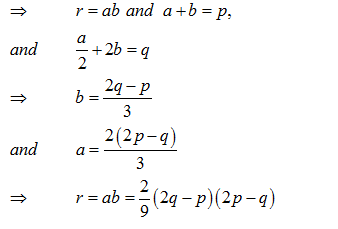Question 5: Let α and β be the roots of

Extra tips for CAT Quadratic Equations Problems
• Where do you study the basic concepts for this problem? The best resource to do so is class eleven and class twelve mathematics books. You might be overwhelmed by the large amount of theory for this topic in these books but then that is the surest way to make sure you have covered the basic concepts for quadratic equation problems in detail.

• In case you are a student from non-engineering background, then you might need to go the extra mile in order to understand this topic. Since quadratic equation is an important topic of +1/+2 algebra, most students preparing for engineering entrance exams go through this topic in detail. This should not scare as these questions asked in MBA entrance exams are generally more generic in nature and do not probe extremely tough mathematical concepts for this topic.

• For quite a few problems for Quadratic Equations, you can use graphs to solve the questions. You should make sure you can use graphs to solve Quadratic Equation problems as this approach can save a lot of time for you while solving questions in the exam.

• For those of you who are scared with Algebra, make sure you take one step at a time and try to learn the basics of Algebraic equations and Linear equations before you approach this topic. Only when you have clear understanding of basic Algebra concepts you will be able to do well in this topic.

Get Posts Like This Sent to your Email
Updates for Free Live sessions and offers are sent on mail. Don't worry: we do not send too many emails..:)
Get Posts Like This Sent to your Email
Updates for Free Live sessions and offers are sent on mail. Don't worry: we do not send too many emails..:)